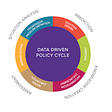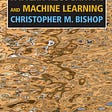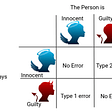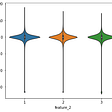# Backorder Prediction With Machine Learning

2. ML Problem formulation
4. Overview of dataset
5. Performance metrics
6. Existing Solutions
7. First Cut Approach
8. Exploratory Data Analysis
9. Data preprocessing
10. Feature Engineering
11. Data Preparation
12. Random Model
13. Machine Learning Models
14. Summary
15. Deployment
16. Future works
17. Code repository
18. References

Backorder is a scenario where a customer can order a product even when we have a lack of product in stock. Backorder should not be confused with out of stock. There are many situations where a backorder can persist.

Unusual demand: Every organization aims to increase the sale of products that they have, Having a poor forecast system can be a reason for such scenarios.

Poor supply chain management: if a problem persists in supply chain management or improper planning, Having improper management of raw material can lead to a problem of poor SCM.

Inventory Management: Not keeping track of inventory and visibility of the inventory might lead to the problem of backorder

The case study “Backorder Prediction” deals with predicting the backorders of products by applying Machine Learning techniques to overcome or reduce the cost of backorders. We will identify parts with the highest chances of shortage so that we could present a high opportunity to improve the company’s overall performance.

# 2. Representing it as an ML problem:

● Our Task is a binary Classification where we have to predict if our product goes on Backorder or not.

○ Yes: If the product goes on Backorder.

○ NO: If the product is not on Backorder.

# 3. Business objectives and constraints

● No Latency requirement

● Retraining is required since it is a time-series data

● Misclassification may result in less effective supply chain management systems such as inaccurate demand forecasting and misclassification of backorder products.

# 4. Overview of Dataset

a. National_inv: Current inventory level of component

b. SKU: Stock Keeping unit

d. Forecast 3,6,9 Months: Forecast sales for the next 3, 6 and 9 months

e. Sales 1,3,6 Months: Sales quantity for the prior 1, 3, 6months

f. Pieces past due: Parts overdue from source

g. Performance: source performance in last 6 and 12 month

h. Local bo_qty: Minimum recommended amount in stock

i. Deck Risk: general risk flag

j. Oe constraints: Amount of stock orders overdue

k. Went_on_backorder : went on backorder or not

The Dataset consists of 15 numerical features and 8 Categorical features along with the target variable.

# 5. Performance metrics

1. This is a Binary classification problem We would be using a confusion matrix as this KPI gives us Many Values Like TP,TN,FP,FN .
2. Since this is an imbalance data set accuracy measure will not be usefull, Instead we can use Precision, Recall, F1 score.
3. Precision = TruePositives / (TruePositives + FalsePositives)
4. Precision is simply the ratio of correct positive predictions out of all positive predictions made, or the accuracy of minority class predictions, But it does not give us an idea about False Negatives.
5. We can use recall to overcome this.

Recall = TruePositives / (TruePositives + FalseNegatives)

The recall is a metric that tells the number of correct positive predictions made out of all positive predictions that could have been made.

F1 Score = (2 * Precision * Recall) / (Precision + Recall)

1. a F1 measure keeps track of both Precision and recall together
2. It is also called the harmonic mean of two fractions
3. Our data is highly imbalanced these KPIs will be useful to monitor our minority class.

# 6. Existing Solutions

This article talks about taking business decisions for the company by predicting backorders with the use of machine learning. The use of machine learning will provide flexibility to the company’s decision-makers which would result in a better and smooth supply chain process. To deal with diverse characteristics of data, this article aims at using ranged methods for specifying different levels of predicted features. This range is tunable and will give flexibility to the decision making authority. Since it is a decision making problem Decision trees based approach can be used in this. This would make the model interpretable without having expert knowledge. The tree-based machine learning algorithm is chosen which includes Random Forest and Gradient Boosting. With the use of a ranged methods approach for the imbalanced dataset, the performance of machine learning has improved by 20%. The data contains monthly, quarterly, half-yearly sales and forecast sales information, inventory level and flag based information. The use of ensemble techniques provided much better results when precision and recall were taken as the performance metrics.

This solution starts with loading the dataset, checking and making observations based on the data stats which include finding a number of features, independent and dependent features, target variables.

The solution then moves on to the EDA which includes making observations based on Univariate and Bivariate Analysis of features. As a part of Data processing, missing values are fixed using Imputation technique like Simple Imputer and MissForest Imputation. Data being highly imbalanced, oversampling techniques like Random Oversample and SMOTE have been used.

As a part of Modelling Random Forest, Adaboost classifier, Stacking Classifier which provides and AUC Score in the range of 0.8 and as a part of Deep learning technique MLP Based classification has been used which provides AUC Score of 0.9.

# 7. First Cut approach

1. My first cut approach would be different from the existing solution on the internet.
2. Finding Correlation between 2 Categorical Features and between 1 Categorical and 1 Numerical Feature.
3. As part of Feature engineering, I am using PCA and SVD for feature extraction and add those features to the existing dataset. Alongside I used a decision tree for discretization for 2 features. i.e Sales and Forecast feature.
4. As a part of modelling, we will try out different models, Logistic regression, Random Forest, GBDT, Stacking Classifier, Custom Ensembles.

# 8. Exploratory Data Analysis(EDA)

The data is Highly imbalanced as we can see the Majority Class i.e is went_on_backorder=No is 99.28 % and went on backorder is 0.72%

1. the bar plot shows the categorical feature rev_stop with the target variable.
2. No order is on backorder if the rev_stop is Yes.
3. similarly, we took oe_constraint with the target variable.
4. when Oe_constraint is set to yes then 0.006 % chance of going on backorder.
5. Deck risk Doesn't seem useful as the proportion for both classes is equal.

6. Also pap risk doesn't seem useful as they have equal proportion.

7. Stop auto-buy also doesn't seem useful it has equal proportion.

# Numerical Features :

1. Since the dataset contained extreme outliers, the Boxplots used for analysis did not offer much information or conclusions about the patterns of data.
2. So I considered taking only the 90th and 80th percentile of the data so that the boxplot might provide us with some information.
1. a. The IQR for the in_transit_qty feature is very small and there are many outliers that did not go in back order
b. The IOR are for both classes are almost overlapping
c. Reduced the data to 90th percentile and the range was dropped to up to 16
d. The box plot of in_transit_qty indicates that if the value is less than 2 then it goes on backorder and if greater than 6 then it did not go on backorder.
2. a. The Boxplot for all three forecast features is almost similar
b. It contains Outliers in all three features
c. if we consider only the 80th percentile of data then it is observed that the forecast is higher than there is a chance of going on backorder.
3. a. The Boxplot for all three sale features is almost similar and also similar to the forecast.
b. It contains Outliers in all three features.
c. if we consider only the 80th percentile of data then it is observed that if the sale is higher then there is a chance of going on backorder.
4. We can see from the boxplot that which underperformed went on backorder.
5. a. From the boxplot we can draw a conclusion that local_bo quantity and pieces past can be ignored as it has only 1 % of non zero data.
b. Most of the data is right Skewed we need to apply feature engineering on it.
c. we can consider only the 90th percentile of the data.
d. Sale and forecast and performance have the same feature so we can select any one group of it.

Bivariate Analysis :

1. We took up the top 5 important features, Since the data is highly imbalanced pair plots are not clear.
2. Forecast and sales features are showing some correlation between each other.
3. A lower forecast for the performance of 6 months shows the chance of items going on backorder.
4. low national_inv with performance up to 0.7 shows the tendency to go on backorder.

# Correlation Matrix

Here is the Correlation matrix

`plt.figure(figsize=(16, 10))sns.heatmap(data.corr(),vmin=-1, vmax=1,annot=True)`

observations :

1. Let's move along Y-axis a. In transit quantity is related to forecast, sales and min bank that is because higher sales, performance and min bank higher the in transit quantity, It ranges from 0.66 to 0.75.
2. As seen in EDA Forecast columns of 3,6,9 have similar distribution so they have interrelated high relation, It has a high co-relation with sales ranging from 0.62 to 0.90.
3. performance is very highly correlated with each other with almost 0.97.
4. Min_bank is highly correlated with sales and forecast as stated earlier.
5. Sales is also high within each other as stated in EDA from 0.82 to 0.98.
6. Pieces pas due is weakly correlated with sales and forecast.
7. national_inv is weakly correlated with min_bank.
8. Linear Models may not perform well as many features are correlated.

# Chi-Square Test:

The Chi-Square test statistical hypothesis is a test for checking Independence between the two categorical variables. The aim is to conclude whether the given two variables are related to each other.

**Null Hypothesis**: There is no correlation between the Categorical feature and target variable.

**Alternate Hypothesis**: There is a correlation between the Categorical feature and target variable.

We can verify the hypothesis using **p-value**.

The significance factor will help us determine whether the given two features have considerable significance. The significant factor is also called as Alpha value.

Usually, an alpha value of 0.05 is chosen.

If the p-value is strictly greater than the alpha value, then H0 ie Null Hypothesis holds true.

Code Snippet:

`def FunctionChisq(Data, Target, List):    from scipy.stats import chi2_contingency        # Creating an empty list of final selected predictors    Predictors=[]        print('##### ChiSq Results ##### \n')    for pred in List:        CrossTab=pd.crosstab(index=Data[Target], columns=Data[pred])        Result = chi2_contingency(CrossTab)                # If the ChiSq P-Value is <0.05, that means we reject H0        if (Result < 0.05):            print(pred, 'is correlated with', Target, '| P-Value:', Result)            Predictors.append(pred)        else:            print(pred, 'is NOT correlated with', Target, '| P-Value:', Result)                        return(Predictors)cat_col=['deck_risk','oe_constraint','ppap_risk','stop_auto_buy','rev_stop']FunctionChisq(Data=data,               Target='went_on_backorder',              List= cat_col)`

Finding Correlation between Categorical and Numerical Features

1. we will be using the ANOVA test for finding relation Between Categorical and Continous features
2. ANOVA Stands for Analysis Of variance, It is used to measure if there is a significant Difference Between the means of the values of the numeric variable for each categorical variable
3. Below items must be remembered about the ANOVA hypothesis test
4. Null hypothesis(H0): The variables are not correlated with each other
5. P-value: The probability of the Null hypothesis being true
6. Accept the Null hypothesis if P-value>0.05. Means variables are NOT correlated
7. Reject Null hypothesis if P-value<0.05. Means variables are correlated

**Null Hypothesis**: There is no correlation between the two features.

**Alternate Hypothesis**: There is a correlation between the two features.

We can verify the hypothesis using **p-value**.

Code Snippet

`def FunctionAnova(Data, Target, List):    from scipy.stats import f_oneway# Creating an empty list of final selected predictors    Predictors=[]        print('##### ANOVA Results ##### \n')    for pred in List:        CategoryLists=Data.groupby(pred)[Target].apply(list)        Results = f_oneway(*CategoryLists)                # If the ANOVA P-Value is <0.05, that means we reject H0        if (Results < 0.05):            print(pred, 'is correlated with', Target, '| P-Value:', Results)            Predictors.append(pred)        else:            print(pred, 'is NOT correlated with', Target, '| P-Value:', Results)        return(Predictors)conti_col=['national_inv','lead_time','in_transit_qty','forecast_3_month','forecast_6_month','forecast_9_month','sales_1_month','sales_3_month',           'sales_6_month','sales_9_month','pieces_past_due','perf_6_month_avg','perf_12_month_avg','local_bo_qty']# Calling the function to check which continous variables are correlated with targetFunctionAnova(Data=data,               Target='went_on_backorder',               List=conti_col)`

# 9. Data Preprocessing:

1. Converting categorical Features Variable to 0 and 1 along with Target variable.
2. Replacing -99 from performance average feature with NaN.
`data.drop(['sku'],axis=1,inplace=True) #since all id are different it does not provide any additional informationcatcol=data.select_dtypes(include = ['object']).columnsfor col in catcol:    data[col].replace({'No': 0, 'Yes': 1}, inplace=True)    data[col] = data[col].astype(int)#Replacing -99 values in performance columns with NaNdata.perf_12_month_avg.replace({-99.0 : np.nan}, inplace = True)data.perf_6_month_avg.replace({-99.0 : np.nan}, inplace = True)`
1. Removing Outlier points
2. Observed from the percentiles that values above 99 percentiles are extremely high and can be termed as an outlier and will be removed as a part of data cleaning.
`national_inv = list(data['national_inv'])national_inv.sort()for i in range(90,101,1):    print(i,'percentile value is',np.percentile(national_inv,i))#for 90 to 100 percentilenational_inv=list(data['national_inv'])national_inv.sort()for i in np.arange(0.0,1.0,0.1):    print('{}percentile value is {}'.format(99+i,national_inv[int(len(national_inv)*(float(99+i)/100))]))print("100 percentile value is ",national_inv[-1])#for 99.0 to 100 percentile`

Percentiles:

3. Here we see that values after 99th percentiles are extremely large. So these rows will be removed from the dataset. Similarly, I have performed for other columns as well.

4. Here we see that we have removed 48564 data points that were classified as outliers.

`df=data[(data.national_inv>= 0.00)&(data.national_inv<=5487.000)&(data.in_transit_qty <=5510.00) & (data.forecast_3_month <=2280.0)\    &(data.forecast_6_month <= 4335.660) &(data.forecast_9_month<=6316.0)&(data.sales_1_month<=693.0)&(data.sales_3_month<=2229.0)&\    (data.sales_6_month<=4410.0)&(data.sales_9_month<=6698.0)&(data.min_bank<=679.6560)]outliers removed : 48564`

5. Train Test Split

`X=df.drop(['went_on_backorder'], axis=1)y=df['went_on_backorder'].valuesX_train, X_test, y_train, y_test = train_test_split(X, y, test_size=0.20, random_state=42)X_train, X_cv, y_train, y_cv = train_test_split(X_train, y_train,random_state = 42 ,stratify=y_train,test_size=0.10)`

Here I am assigning Target Variable to another variable and dropping the Target variable and SKU columns.

# 10 Feature Engineering

1. Model-Based Imputation(KNN Imputer): we will use model-based imputation for imputing the missing values.
2. we set neighbour = 5 and replace all the missing values
`imputer = KNNImputer(n_neighbors=5)df_train_imputed = pd.DataFrame(imputer.fit_transform(X_train),columns = X_train.columns)df_cv_imputed= pd.DataFrame(imputer.transform(X_cv),columns = X_cv.columns)df_test_imputed=pd.DataFrame(imputer.transform(X_test),columns = X_test.columns)`

3. PCA: PCA stands for Principal component analysis. a statistical procedure that uses an orthogonal transformation that converts a set of correlated variables to a set of uncorrelated variables.

4. PCA is the most widely used tool in exploratory data analysis and in machine learning for predictive models.

`pca=PCA(n_components=2)train_pca=pca.fit_transform(df_train_imputed)cv_pca=pca.transform(df_cv_imputed)test_pca=pca.transform(df_test_imputed)for i in range(2): #for adding the feature to df    df_train_imputed['PCA'+str(i)]=train_pca[:,i]    df_test_imputed['PCA'+str(i)]=test_pca[:,i]    df_cv_imputed['PCA'+str(i)]=cv_pca[:,i]`

5. Truncated SVD

SVD stands for Single Value Decomposition. SVD can be thought of as a projection method where data with n-columns(features) is projected into a subspace with n or less than n columns, whilst retaining the essence of original data.

SVD is used widely both in the calculation of other matrix operations such as matrix inverse as well as a data reduction method in machine learning.

`svd = TruncatedSVD(n_components=2)train_svd=svd.fit_transform(df_train_imputed)cv_svd=svd.transform(df_cv_imputed)test_svd=svd.transform(df_test_imputed)for i in range(2): #for adding the feature to df    df_train_imputed['SVD'+str(i)]=train_svd[:,i]    df_test_imputed['SVD'+str(i)]=test_svd[:,i]    df_cv_imputed['SVD'+str(i)]=cv_svd[:,i]`

6. Feature Binning and Discretization of using Decision Trees.

Discretization is a process of transforming continuous variables into discrete variables by creating a set of contiguous intervals that span the range of variable values. This helps us handle outliers by placing them into lower or higher intervals. These outlier observations no longer differ from the rest of the values at the tail distributions as they are in the same interval.

Using Discretization also helps spread the skewed variables across a set of bins of equal observation numbers.

Discretization with Decision Trees consists of using Decision Tree to identify optimal splitting points that would determine bins.

`score_ls = []     # here I will store the roc aucscore_std_ls = [] # here I will store the standard deviation of the roc_aucfor tree_depth in [1,2,3,4,5,7,8,9,10]:    tree_model = DecisionTreeClassifier(max_depth=tree_depth)        scores = cross_val_score(tree_model, df_train_imputed.sales_9_month.to_frame(),           y_train, cv=3, scoring='roc_auc')           score_ls.append(np.mean(scores))        score_std_ls.append(np.std(scores))    temp = pd.concat([pd.Series([1,2,3,4,5,7,8,9,10]), pd.Series(score_ls), pd.Series(score_std_ls)], axis=1)temp.columns = ['depth', 'roc_auc_mean', 'roc_auc_std']print(temp)tree_model = DecisionTreeClassifier(max_depth=4)tree_model.fit(df_train_imputed.sales_9_month.to_frame(), y_train)df_train_imputed['sales_9_tree']=tree_model.predict_proba(df_train_imputed.sales_9_month.to_frame())[:,1]df_test_imputed['sales_9_tree']=tree_model.predict_proba(df_test_imputed.sales_9_month.to_frame())[:,1]df_cv_imputed['sales_9_tree']=tree_model.predict_proba(df_cv_imputed.sales_9_month.to_frame())[:,1]`

# Main Conclusion from EDA and Feature Engineering:

1. It is a binary classification with high imbalance Data
2. Data consists of both Numerical and categorical Data
3. Missing values are in the Lead_time features and performance 6 & 12 months columns consist of -99 which is replaced by NaN
4. Almost all the numerical columns had extreme skewness (on the positive side) indicating them as outliers or also can be useful data as sale, inventory, forecast of some products might be very high
5. Categorical columns consist of yes or no
6. As a part of preprocessing and Feature engineering we will drop the SKU column and also we dropped the last row, We also combined train and test data together and then split it into 80:20 ratio using train test split
7. encoded target variable and dependent variable with No as 0 and Yes as 1
8. Took only 99 % of data since the data after 99 showed some high values
8. Imputed missing values with KNN imputer and stored it as imputer.csv file so that we need not run it again and again
9. We used SVD and PCA for dimensionality reduction
10. Performed Hypothesis test on different features to find co-relation between target and all variables
11. we got 5 important features using RF and feature extraction methods
11. Used bining as a feature engineering technique where i tried bining top 5 features and found forecast_9 and sales_9 added some value
12. https://towardsdatascience.com/discretisation-using-decision-trees-21910483fa4b referred to this blog, I performed decision tree-based bining where I did hyperparameter tuning to get the optimal value to bin the feature accordingly.

# 11. Data Preprocessing :

for Data processing we will apply the min-max scalar to scale the data between 0–1

`scaler=MinMaxScaler()df_train_imputed=pd.DataFrame(scaler.fit_transform(df_train_imputed),columns=df_train_imputed.columns)df_cv_imputed=pd.DataFrame(scaler.transform(df_cv_imputed),columns=df_cv_imputed.columns)df_test_imputed=pd.DataFrame(scaler.transform(df_test_imputed),columns=df_test_imputed.columns)`

# Random Model

Before we start with Machine Learning models, it would be good to test the dataset on reference models and we will take this random model as a benchmark for the ML models. All other models should perform better than Random Model. So here we are making a random model on the dataset.

`random_clf = DummyClassifier(strategy="uniform")random_clf.fit(df_train_imputed, y_train)print("ROC-AUC score  : " , roc_auc_score(y_test,random_clf.predict_proba(df_test_imputed)[:, 1]))print("Macro F1-Score : " , f1_score(y_test,random_clf.predict(df_test_imputed),pos_label = 1,average = 'macro'))ROC-AUC score  :  0.5Macro F1-Score :  0.33950438459968`

This model randomly generates 1s and 0s as outputs and gives a macro f1-score of 0.33 on the test.

# 13. Machine Learning Models

As the data is imbalanced it would be better to go for Tree-based models such as Random Forest, Adaboost classifier, GBDT. Initially, I will be applying Logistic Regression. As a part of Custom Ensembles, I will be using Decision Tree as a base model and Logistic Regression as Meta Classifier. Also, we will be using a Stacking classifier.

Logistic Regression

Logistic regression is a classification algorithm based on a given set of independent variables. It is used to estimate discrete values ie 0s and 1s. Basically, it measures the relationship between the categorical dependent variable and set of independent variables by estimating the probability of occurrence of an event using its logistic function.

Using various values of alpha as a hyperparameter…

`Best Params :  {'C': 100, 'penalty': 'l2'}Best Score :  0.8991768267468859`

This provided a test F1-Score of 0.47 on the oversampled dataset and 0.49 on data.

Random Forest

Random Forest is an extension to Decision Trees. It uses a bagging strategy that involves Bootstrap and Aggregation. In this modelling technique, several Decision Trees are trained on the train data and a majority vote is taken between these many decision trees for classifying the points.

Used GridSearchCV for hyperparameter tuning and the best model was found to be of max_depth=50, min_samples_split=5, n_estimators=500 for dataset.

Test F1-Score was 0.62 on the dataset.

An AdaBoost classifier is a meta-estimator that begins by fitting a classifier on the original dataset and then fits additional copies of the classifier on the same dataset but where the weights of incorrectly classified instances are adjusted such that subsequent classifiers focus more on difficult cases.

Used GridSearchCV for hyperparameter tuning and the best model was found to be of n_estimators=1000 for the dataset.

Test F1-Score was 0.53 on the dataset.

GBDT

Gradient Boosted Decision Trees (GBDT) is a machine learning algorithm that iteratively constructs an ensemble of weak decision tree learners through boosting.

Used GridSearchCV for hyperparameter tuning and the best model was found to be of max -depth=5,n_estimators=500 for the dataset.

Test F1-Score was 0.56 on the dataset.

Stacking Classifier

Model stacking is an efficient ensemble method in which the predictions, generated by using various machine learning algorithms, are used as inputs in a second-layer learning algorithm. This second-layer algorithm is trained to optimally combine the model predictions to form a new set of predictions.

we stacked all the above-mentioned models and used logistic regression as a meta-classifier.

Test F1-Score was 0.55 on the dataset.

Custom Ensembles

We are using ’n’ different Decision Trees and Training them with randomly sampled data with repetition. The output of the decision trees is provided as input to the meta classifier (Logistic Regression). we also used median imputed data for the missing values

The entire dataset is being split into D1, D2 and Test data with a ratio of 40:40:20. D1 is randomly sampled. We are oversampling the randomly sampled dataset by sampling it with repetition. This data is used to train Decision Trees.D2 is passed on the trained Decision Trees to obtain the output. Now, this output is used to train Meta Classifier(Logistic Regression) to obtain final output and GridSearchCV is performed on this to obtain the best f1-score. This was performed on data with FE and without FE.

The Test F1 score is 0.61 on the dataset.

# 14. Summary

We observe that with every model the improvement in f1 scores.

1. Logistic regression is least performing from all
2. Random forest shows a good score with an F1 score of 0.63
3. Adaboost shows better performance than RF and it has an F1 score greater than RF(0.53)
4. GBDT is performing well with an F1 score up to 0.56
5. Stacking classifiers also show similar performance but slightly lower than GBDT
6. Custom Ensembles with DecisionTree Regressor with an F1 score of 0.62

# 15. Deployment

The case study was deployed on the local system with a basic HTML page and Flask Server. There is a download link provided to download the dataset in the form of a text file.

# 16 Future Works

• As a part of Future works, we can implement Deep learning models on to this to check the performance of models.
• we can also do over and undersampling with robust scaling.
• We can also include Polynomial Featurization as a part of Feature Engineering to check the performance of models.

# 17 . Code Repository

The code related to case study can be found in the below link

# 18. References

--

--

--

## More from shubham dahiwalkar

Love podcasts or audiobooks? Learn on the go with our new app.

## Better Data for Better Policy: Accessing New Data Sources for Statistics Through Data…## Pattern Recognition and Machine Learning## Python Set Difference — A Complete Beginner Guide## Predicting butter prices## The Big Data: Trouble and Advantage for the Big firms## Pie Sales Prediction Using Multiple Linear Regression in Python## AI safety, AI ethics and the AGI debate## P Value, Significance Level, Confidence Interval and Confidence Level## Elo Merchant Category Recommendation-A case study## Correct your writing with machine translation## Hyper-Automation, UiPath AI-Center & Sentiment Analysis with possible Use-Cases